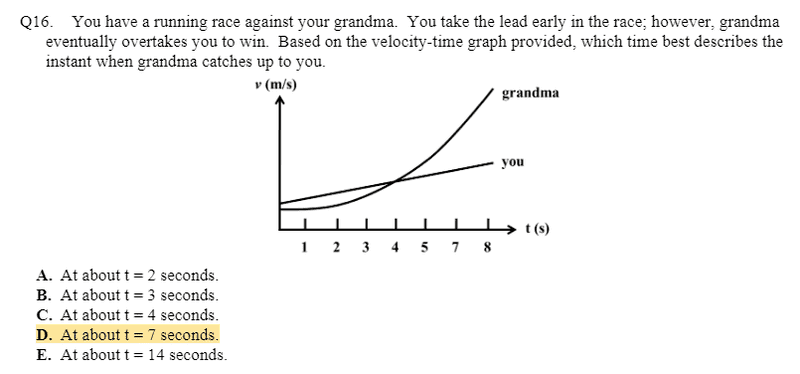# Predicting Position for velocity time graph

## Homework Statement## The Attempt at a Solution

I honestly have no clue as to how to go about this question. Am i supposed to find the area under the curve? but how do i do that without y axis numbers. am i supposed to look at the slopes?

TSny
Homework Helper
Gold Member
Am i supposed to find the area under the curve? but how do i do that without y axis numbers. am i supposed to look at the slopes?
You only need to estimate the areas close enough to decide among the possible answers.

Buzz Bloom
Gold Member
Hi querty:

I first note that the values given along the X-axis omits 6. I assume that is is a typo, and that the last two ticks are for 6 and 7 rather than 7 and 8. I also note that none of the 5 given answers matches my estimate of the correct time.

The question is: at what point in time is the distance from the starting point the same for the two graphs. Your idea about area is good. Hint: You are not being asked to find the distance corresponding to the desired time.

Hope this helps.

Regards,
Buzz

Hi querty:

I first note that the values given along the X-axis omits 6. I assume that is is a typo, and that the last two ticks are for 6 and 7 rather than 7 and 8. I also note that none of the 5 given answers matches my estimate of the correct time.

The question is: at what point in time is the distance from the starting point the same for the two graphs. Your idea about area is good. Hint: You are not being asked to find the distance corresponding to the desired time.

Hope this helps.

Regards,
Buzz
Okay so I would calculate :
area of me = 0.5 b * h
area of grandma = 0.5 bh + lw ( split the curve into triangle and rectangle )

would i then : area of me - area of grandma ?

Buzz Bloom
Gold Member
Hi querty:

Since you have no numbers for the Y-axis, I suggest you just eyeball it. Imagine a vertical line at the time T when the areas are the same. Estimate the area of grandma minus me, that is the area between the curve and the line. Between the beginning of the graph and the point P when the two graphs cross is area A. Between P and T the area between the graphs is area B. The total area between the beginning and T under grandma will equal the total area under me. How will A and B relate to each other?

Regards,
Buzz